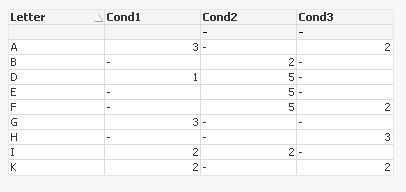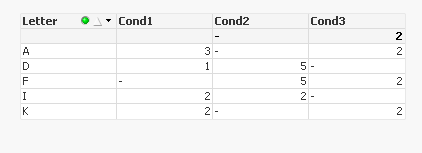# QlikView App Development

Discussion Board for collaboration related to QlikView App Development.

Announcements
Modernize Your QlikView Deployment webinar, Nov. 3rd. REGISTER
cancel
Showing results for
Did you mean:
HighlightedCreator III

## counting when 2 or 3 conditions are not null

whereand I would like to show only rows where 2 or 3 of the columns are not null (in other words to exclude rows with 2 or more null)

so expecting to see:using Sunny's solution I am Now trying to count in a Text Object how many lines are matching 2 or 3 conditions - so how many are in the solution: here for example A+D+F+I+K = 5

so I will expect to see 5 in the text Object.

Any idea,

Thanks.

Raphael

1 Solution

Accepted Solutions
HighlightedMVP

May be try this

=Count(DISTINCT If(RangeSum(If(Len(Trim(Cond1)) = 0, 1), If(Len(Trim(Cond2)) = 0, 1), If(Len(Trim(Cond3)) = 0, 1)) < 2, Letter))

or this

=Count(DISTINCT {<Letter = {"=RangeSum(If(Len(Trim(Cond1)) = 0, 1), If(Len(Trim(Cond2)) = 0, 1), If(Len(Trim(Cond3)) = 0, 1)) < 2"}>} Letter)

3 Replies
HighlightedMVP

May be try this

=Count(DISTINCT If(RangeSum(If(Len(Trim(Cond1)) = 0, 1), If(Len(Trim(Cond2)) = 0, 1), If(Len(Trim(Cond3)) = 0, 1)) < 2, Letter))

or this

=Count(DISTINCT {<Letter = {"=RangeSum(If(Len(Trim(Cond1)) = 0, 1), If(Len(Trim(Cond2)) = 0, 1), If(Len(Trim(Cond3)) = 0, 1)) < 2"}>} Letter)

HighlightedCreator III

Both working perfect, many thanks

HighlightedMVP

I would use the second expression if both of them works